GCSE Maths Algebra Inequalities

Greater Than Sign

# Greater Than Sign

Here we will learn about the greater than sign including the symbol used to represent it, other comparison symbols and comparing numbers and expressions using the greater than sign.

There are also inequality worksheets based on Edexcel, AQA and OCR exam questions, along with further guidance on where to go next if you’re still stuck.

## What is the greater than sign?

The greater than sign is a mathematical symbol used to compare numbers and expressions. Otherwise known as a comparison symbol.

The greater than sign is

The greater than sign is also known as the more than sign. The wide end of the symbol always faces the bigger number or expression – the symbol looks open towards the bigger number and ‘points’ at the smaller value like an arrow.

For example,

This is read as ‘10 is greater than 6’.

15>10 is read as ‘15 is greater than 10’.

2.3>1.8 is read as ‘2.3 is greater than 1.8’.

The greater than sign is also used to represent inequalities in maths.

For example, x>2   is read as  ‘x is greater than 2’.

Step-by-step guide: Inequalities

There are more comparison symbols (or inequality symbols) you need to know.

• Equal to = using the equals sign denotes when two values are exactly equal.

For example, 3+4=7.

• Not equal to this symbol means two values are definitely not equal.

For example, 3+4 10.

• Identical to \equiv denotes when two values are identical.

For example, 4(x+2)\equiv 4x+8.

• Less than < denotes when one value is smaller than another.

For example, 3<7.

Step-by-step guide: Less than sign (coming soon)

• Less than or equal to \le denotes when we know a value is smaller or equal to.

• Greater than or equal to \ge denotes when we know a value is bigger or equal to.

### What is the greater than sign?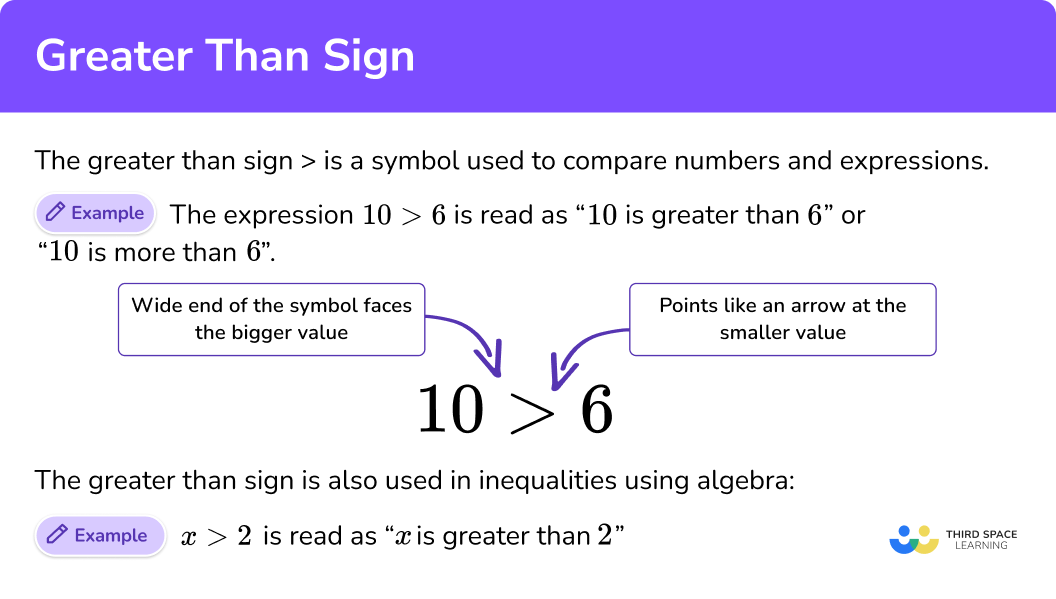## How to compare values using the greater than sign

In order to compare values using the greater than sign:

1. Compare the values given.
2. If greater than, write the larger value on the left hand side.
3. If greater than, write the smaller value on the right hand side.
4. Write the values with the correct symbol, or place the numbers on the correct sides of a given symbol.

### Explain how to compare values using the greater than sign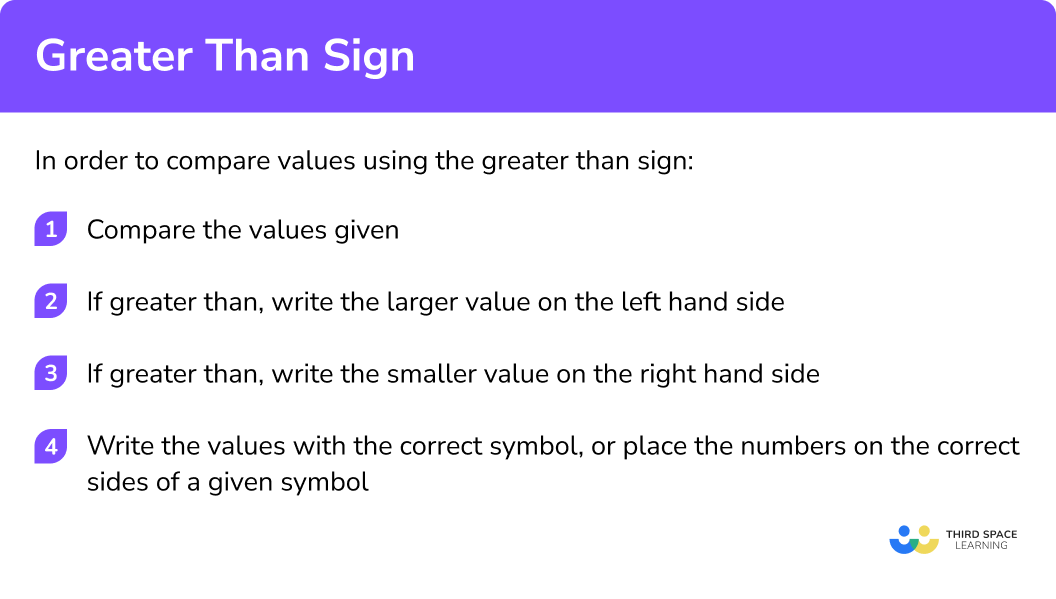## Related lessons on inequalities

Greater than sign is part of our series of lessons to support revision on inequalities. You may find it helpful to start with the main inequalities lesson for a summary of what to expect, or use the step by step guides below for further detail on individual topics. Other lessons in this series include:

## Greater than sign examples

### Example 1: comparing numbers

Write the correct sign, > or < , in the box.

1. Compare the values given.

15 is positive, -20 is negative.

So, 15 is the larger of the two values.

2If greater than, write the larger value on the left hand side.

15 is the greater number, and is written on the left side, so we draw the greater than symbol with the open end next to the larger value.

3If greater than, write the smaller value on the right hand side.

-20 is the smaller number, and is written on the right side, so the greater than symbol is pointing to it like an arrow.

4Write the values with the correct symbol, or place the numbers on the correct sides of a given symbol.

### Example 2: comparing numbers

Write the correct sign, > or < , in the box.

Compare the values given.

If greater than, write the larger value on the left hand side.

If greater than, write the smaller value on the right hand side.

Write the values with the correct symbol, or place the numbers on the correct sides of a given symbol.

### Example 3: comparing fractions

Which is larger, \frac{3}{5} or \frac{2}{7}?

Compare the values given.

If greater than, write the larger value on the left hand side.

If greater than, write the smaller value on the right hand side.

Write the values with the correct symbol, or place the numbers on the correct sides of a given symbol.

### Example 4: comparing fractions

Which is larger, 2 \frac{2}{5} or 3 \frac{1}{4}?

Compare the values given.

If greater than, write the larger value on the left hand side.

If greater than, write the smaller value on the right hand side.

Write the values with the correct symbol, or place the numbers on the correct sides of a given symbol.

### Example 5: comparing expressions

Which is larger, 28 \times 7 or 153+38?

Compare the values given.

If greater than, write the larger value on the left hand side.

If greater than, write the smaller value on the right hand side.

Write the values with the correct symbol, or place the numbers on the correct sides of a given symbol.

### Example 6: comparing expressions

Which is larger, 8(x+2) or 2(4 x+14)?

Compare the values given.

If greater than, write the larger value on the left hand side.

If greater than, write the smaller value on the right hand side.

Write the values with the correct symbol, or place the numbers on the correct sides of a given symbol.

### Common misconceptions

• Confusing the greater than and less than symbols

The biggest mistake is writing the symbols the wrong way round. Remember, the sign should point at the smallest number like an arrow.

For example, 14>7, \ ‘14 is greater than 7’, or 7<14, ‘7 is less than 14’.

• Equivalent fractions

When comparing fractions, we have to convert them into equivalent fractions with common denominators, so we can compare the numerators.

### Practice greater than sign questions

1. Which is larger, \frac{4}{7} or \frac{4}{9}? Write your answer using the greater than comparison symbol.

\frac{4}{9} > \frac{4}{7}\frac{4}{9} < \frac{4}{7}\frac{4}{7}>\frac{4}{9}\frac{4}{7} < \frac{4}{9}Convert the fractions to equivalents with a common denominator.

\frac{4}{7}=\frac{36}{63}

\frac{4}{9}=\frac{28}{63}

Comparing the numerators tells us that \ \frac{36}{63}>\frac{28}{63} \ so \ \frac{4}{7}>\frac{4}{9}.

2. Which is larger, 10 or -18? Write your answer using the greater than comparison symbol.

10 > -1810 < -18-18>-10-18<1010 is positive and -18 is negative, so 10 is greater than -18.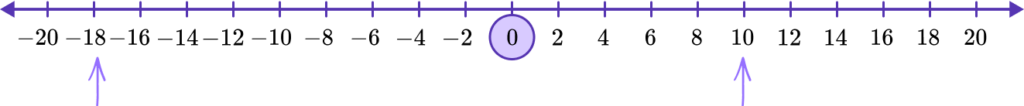3. Which is larger, 2 \frac{3}{5} or 2 \frac{3}{8}? Write your answer using the greater than comparison symbol.

2 \frac{3}{5}<2 \frac{3}{8}2 \frac{3}{5}>2 \frac{3}{8}2 \frac{3}{8}>2 \frac{3}{5}2 \frac{3}{8}<2 \frac{3}{5}Convert the fractions to equivalents with a common denominator.

2 \frac{3}{5}=\frac{104}{40}

2 \frac{3}{8}=\frac{95}{40}

Comparing the numerators tells us that \ \frac{104}{40}>\frac{95}{40} \ so \ 2 \frac{3}{5}>2 \frac{3}{8} .

4. Which is larger, 8 \times 18 or 12 \times 13? Write your answer using the greater than comparison symbol.

8 \times 18>12 \times 138 \times 18<12 \times 1312 \times 13<8 \times 1812 \times 13>8 \times 18Evaluating each expression gives,

8 \times 18=144

12 \times 13=156

So 12 \times 13>8 \times 18.

5. Which is larger, 10 \% of 2890 or 5 \% of 4568? Write your answer using the greater than comparison symbol.

10 \% of 2890<5 \% of 456810 \% of 2890>5 \% of 45685 \%  of 4568<10 \% of 28905 \% of 4568>10 \% of 2890Evaluating each expression gives,

10 \% of 2890=289

5 \% of 4568=228.4

So, 10 \% of 2890>5 \% of 4568.

6. Which is larger, 6(x+3) or 3(2x+4)? Write your answer using the greater than comparison symbol.

6(x+3)>3(2x+4)6(x+3)<3(2 x+4)3(2x+4)>6(x+3)3(2x+4)<6(x+3)Evaluating each expression gives,

6(x+3)=6x+18

3(2x+4)=6x+12

So, 6(x+3)>3(2x+4).

### Greater than sign GCSE questions

1. Write the correct sign >, =, or in each box.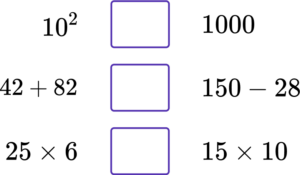(3 marks)

10^2 1000

(1)

42+82>150-28

(1)

25\times 6=15\times 10

(1)

2. Here are four number cards.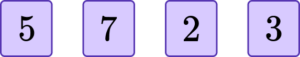Arrange the cards to give a possible expression below.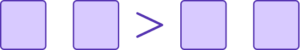(1 mark)

Any correct expression where the LHS is greater than the RHS.

For example, 57>23.

(1)

3. Here are two fractions.

Which fraction is greater?

Complete the inequality expression below.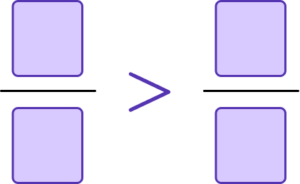(2 marks)

\frac{7}{8}=\frac{28}{32} \ or \ \frac{7}{9}=\frac{24}{32}

(1)

\frac{7}{8}>\frac{3}{4}

(1)

## Learning checklist

You have now learned how to:

• Recognise the greater than sign
• Compare numbers and expressions
• Write expressions using the greater than sign

## Still stuck?

Prepare your KS4 students for maths GCSEs success with Third Space Learning. Weekly online one to one GCSE maths revision lessons delivered by expert maths tutors.

Find out more about our GCSE maths tuition programme.